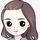cancel
Showing results for
Did you mean:Anonymous
Not applicable

## Get a value from the last row for the student

I am new to Power BI and I am trying to get the last test value for a student where the test could have been taken multiple times.  I need to omit the student if they only took the test once.  There are row numbers per student in the table.  The student number field is UMPI_NBR.  the value I want is PHQ9_TOTAL and the row number field is TESTROW.  All I am pulling right now is the largest value of all of the tests beyond the 1st one instead of the value of PHQ9_TOTAL of the MAX TESTROW.

Last Visit = SUMX (
VALUES( Table1[UMPI_NBR] ),
CALCULATE (MAX(Table1[PHQ9_TOTAL] ), (Table1[TESTROW] > 1) )
)
1 ACCEPTED SOLUTIONAnonymous
Not applicable

This is working for me.  I don't understand the syntax well enough to know why I don't need the UMPI_NBR specified in there somewhere.

Last Visit = CALCULATE (
SELECTEDVALUE ( Table1[PHQ9_TOTAL] ),
FILTER ( ALL ( Table1[VISIT] ), Table1[VISIT] = MAX ( Table1[VISIT] ) && Table1[VISIT] > 1)
)
10 REPLIES 10Anonymous
Not applicable

This is working for me.  I don't understand the syntax well enough to know why I don't need the UMPI_NBR specified in there somewhere.

Last Visit = CALCULATE (
SELECTEDVALUE ( Table1[PHQ9_TOTAL] ),
FILTER ( ALL ( Table1[VISIT] ), Table1[VISIT] = MAX ( Table1[VISIT] ) && Table1[VISIT] > 1)
)Super User

Hi,

What do you mean by "I need to omit the student if they only took the test once"?  Share a dataset and show the expected result.

Regards,
Ashish Mathur
http://www.ashishmathur.comCommunity Support

Hi @Anonymous ,

I made a minor adjustment on the formula you provided , and now you should get the correct value, please check.Last Visit = VAR a =    MAX ( 'Table1'[UMPI_NBR] )VAR b =    SUMX (        VALUES ( Table1[UMPI_NBR] ),        CALCULATE (            MAX ( Table1[PHQ9_TOTAL] ),            DISTINCTCOUNT ( Table1[TESTROW] ) > 1                && 'Table1'[UMPI_NBR] = a        )    )RETURN    b

Best Regards

Rena

Community Support Team _ Rena
If this post helps, then please consider Accept it as the solution to help the other members find it more quickly.Anonymous
Not applicable

This answer gives me the next test score after the 1st one but not necessarily the last one.Super User

Try like

``````//1
Last Visit = SUMX (
VALUES( Table1[UMPI_NBR] ),
CALCULATE (MAX(Table1[PHQ9_TOTAL] ), filter(Table1,Table1[TESTROW] = max(Table1[TESTROW])) ))

//2
rank = rank(Table1[UMPI_NBR],calculate(max(Table1[TESTROW])),,desc,dense)

Last Visit = SUMX (filter(Table1,[rank]=1),Table1[PHQ9_TOTAL] )
``````

Appreciate your Kudos. In case, this is the solution you are looking for, mark it as the Solution.
In case it does not help, please provide additional information and mark me with @

Thanks. My Recent Blogs -Decoding Direct Query - Time Intelligence, Winner Coloring on MAP, HR Analytics, Power BI Working with Non-Standard TimeAnd Comparing Data Across Date RangesAnonymous
Not applicable

The 1st answer is close but I need to exclude the 1st score if the person hasn't taken a 2nd test.

The 2nd answer gives me syntax errors at '(' and most of the fields are highlighted as errors.Community Support

Hi @Anonymous ,

In fact , the formula I provided works well in my side... Here is the sample PBIX file I used , please check if there is any diffrence between yours and mine...

If I understand correctly , what you want to get is the total score of the best results of those students that took the test of multiple times. So I got the result by the following steps :

1 . Exclude students that take the test only once

2 . Get the best results from / of students that took the test of multiple times

3 . Summarize the scores obtained above .

For example , the following example contains the test scores of 5 students (S01 ~ S05 ) . Student S01 and S04 took the test only once , so the score of this two students are excluded . In addition , the best scores of S2 , S3 , and S5 are 89 , 82 , and 65 separately . Then the final summarized score is 89 + 82 + 65 = 325 .Best Regards

Rena

Community Support Team _ Rena
If this post helps, then please consider Accept it as the solution to help the other members find it more quickly.Anonymous
Not applicable

Hi @yingyinr , @Ashish_Mathur , @amitchandak

1.  Yes, I want to exclude the score of the students that took the test only once because one of the things I will be using the score for is to check the effectiveness of a training program.  So I need to know if the score improved or not.  So the ones that have only taken the test once, need to be excluded.

2. I want only the LAST score, not the best one and not a summary.  So if student 'A' took the test 6 times:

TESTROW/PHQ9_TOTAL

1                               80

2                               85

3                              70

4                              80

5                              90

6                              68

I need the #6 - 68Super UserSuper User

Please explain what worked, what did not with you formula and example.